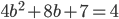Homework #100
Learning Target #47: I can solve quadratic equations by using the quadratic formula.
Class Number *
Your answer
First Name *
Your answer
Last Name *
Your answer
email address *
Your answer
Are you also in Mrs. Hurley's 5th Hour Algebra Lab? *
Solve each equation using the quadratic formula
Use notes on IAN Page 128 to help you.
a)b= *
Your answer
or b= *
Your answer
b)n= *
Your answer
or n= *
Your answer
Use the first ten minutes of class tomorrow to ask any questions you have about the homework, or to clear up any confusion.
Never submit passwords through Google Forms.